Journal of Vibroengineering

Published: 15 February 2019

# Natural vibration of tapered rectangular plate with exponential variation in non homogeneity

Amit Sharma1
Naveen Mani2
Reeta Bhardwaj3
1, 3Amity University Haryana, Gurgaon, India
2Sandip University, Nasik, Maharashtra, India
Corresponding Author:
Amit Sharma
Views 92

#### Abstract

In this paper, authors studied the natural vibration of tapered non homogeneous rectangular plate on clamped edges. For tapering in plate, authors considered circular variation in thickness and for non-homogeneity (in plate’s material) Poisson’s ratio varies exponentially. Bilinear temperature (linear along both the axes) variation on the plate is being viewed. Rayleigh Ritz method is used to solve differential equation of motion. All the results are presented with the help of tables and graphs. A comparison of results is also given to support the present study.

## 1. Introduction

The non uniform and non homogeneous rectangular plate are used in various engineering structures such as aerospace engineering, marine engineering, ocean engineering, optical instruments and mechanical engineering. Non uniform and non homogeneous plates have greater efficiency when compared to uniform and homogeneous plates because of reduction in weight, high tensile strength, durability, elastic behavior and size. The study of plate vibration without consideration of temperature is nothing because almost all engineering structure worked under great influence of temperature. Thus, to design an accurate structure, determination of natural frequencies and mode shape are essential. A significant work has been provided in these directions.

Timoshenko and Woinowsky-Krieger  discussed comprehensive background on plate and shells theory in their book and explained bending (pure and symmetrical) of long, circular, anisotropic and plates having lateral loads, forces in the middle. They also studied rectangular plates (on simply supported and on various edge conditions), plates on elastic foundation and plates of various shapes. Bhat  provided natural frequencies of rectangular plates on clamped, simply supported and combination of simply supported and free edges by applying beam characteristic orthogonal polynomials in the Rayleigh Ritz method and showed that when some edges of plate are free, the present method acquires superior result for lower modes. Chakraverty and Petyt  discussed transverse vibration of elliptic and circular plates on clamped, simply supported and free boundary using two-dimensional boundary characteristic orthogonal polynomials in Rayleigh Ritz method and evaluated first five natural frequencies for various combinations of non homogeneity and aspect ratios of the ellipse. Li  applied Rayleigh Ritz method to analyze the modal characteristics of a rectangular plate with general elastic supports alone its edges and provided numerical examples to demonstrate the accuracy and convergence of the current solution. Ranji and Shahbaztab  analyzed free vibration of non homogeneous and orthotropic plate resting on a Pasternak type of elastic foundation by using Rayleigh Ritz method and provided the effect of foundation, density and non homogeneity parameters on natural frequency. Singh and Sexena  applied Rayleigh Ritz method to study the transverse vibrations of tapered skew plates with different combinations of boundary conditions and obtained first three modes of frequency and mode shapes. Sharma et al. [7, 8] studied the natural vibration of tapered (exponential and circular variation in thickness) square plate with non homogeneity (variation in density and Poisson’s ratio) under temperature variation (bi linear and bi parabolic). An effect of circular variation in thickness on vibrational frequencies of parallelogram plate has been studied by Sharma  and obtained first two modes of vibrations for different combinations of plate parameters on clamped edges. Sharma et al.  also studied the vibration of tapered rectangular plate with linear variation in density and evaluated first two modes of vibration on clamped edges. Khanna and Kaur [11, 12] analyzed the free vibration of non uniform (linear and exponential variation in thickness) and non homogeneous (exponential variation in Poisson’s ratio) of rectangular plate with temperature (linear and exponential) variation by using Rayleigh Ritz method on clamped boundary condition. Hwu  presented closed-form solution for the free vibration problems, to study the effects of rotary inertia and shear deformation on frequency and provided numerical examples which shows the importance of considering the effects of rotary inertia and shear deformation. Avalos and Laura  studied the transverse vibrations of isotropic, orthotropic and anisotropic rectangular plates with two rectangular cutouts on simply supported edge using Classical plate theory. Shufrin and Eisenberger  presented a new semi-analytical method for modeling rectangular plates (with one dimensional variable thickness and cutouts) to analyze the free vibration of rectangular plates with various rectangular cutouts and variable thickness. In order to show the accuracy and convergence of the solution, they compared their results with other semi-analytical methods. Sakiyama and Huang  presented an approximate method to analyze the free vibration of thin and moderately thick rectangular plates with arbitrary variable thickness using Green function and showed that the numerical solution for the Green function has good convergence and accuracy. Wang and Zu  studied the vibrations of functionally graded material (FGM) rectangular plates with porosities and moving in thermal environment by using von Kármán nonlinear plate theory and obtained vibration characteristics such as natural frequency and nonlinear frequency response. Huang et al.  developed a discrete method to analyze the free vibration problem of orthotropic rectangular plates with variable thickness using Green function and obtained the effects of the aspect ratios, boundary conditions and the variation of the thickness on the frequencies. Huang et al.  proposed new method (FBGM) to analyze the free vibration of cantilever plates with variable thickness and obtained the solution of characteristic equation of free vibration. Taher et al.  evaluated first nine frequency parameters of circular and annular plates with variable thickness and combined boundary conditions, for different thickness to radius ratios by using three-dimensional elasticity theory. Zur  discussed the free vibration of homogeneous and isotropic annular thin plates with variable distributions of parameters by using Green's function and Neumann series, and obtained numerical solutions of the characteristic equations for constant and hyperbolic varying thickness on different boundary conditions. Zur  discussed the free vibration analysis of homogeneous and isotropic circular thin plates with variable distribution of parameters by using Green's functions and evaluated six lower natural dimensionless frequencies of axisymmetric vibration of circular plates of constant and variable thickness.

In this study, authors show the effect of circular variation in thickness on free vibration of non homogeneous rectangular plate with linear (along both the axes) variation in temperature and compute the vibrational frequency modes (first two modes). The results are presented with the help of tables and figures.

## 2. Differential equation of motion

The differential equation for transverse motion of the plate is:

1
$\frac{{\partial }^{2}{M}_{\zeta }}{\partial {\zeta }^{2}}+2\frac{{\partial }^{2}{M}_{\zeta \psi }}{\partial \zeta \partial \psi }+\frac{{\partial }^{2}{M}_{\psi }}{\partial {\psi }^{2}}=\rho l\frac{{\partial }^{2}\varphi }{\partial {t}^{2}},$

where:

2

Using Eq. (2), Eq. (1) becomes:

3
$\left[{D}_{1}\left(\frac{{\partial }^{4}\varphi }{\partial {\zeta }^{4}}+2\frac{{\partial }^{4}\varphi }{\partial {\zeta }^{2}\partial {\psi }^{2}}+\frac{{\partial }^{4}\varphi }{\partial {\psi }^{4}}+\frac{{\partial }^{2}\nu }{\partial {\zeta }^{2}}\frac{{\partial }^{2}\varphi }{\partial {\psi }^{2}}\right)+2\frac{\partial {D}_{1}}{\partial \zeta }\left(\frac{{\partial }^{3}\varphi }{\partial {\zeta }^{3}}+\frac{{\partial }^{3}\varphi }{\partial \zeta \partial {\psi }^{2}}+\frac{\partial \nu }{\partial \zeta }\frac{{\partial }^{2}\varphi }{\partial {\psi }^{2}}\right)\right$

For solution of Eq. (3), we can take deflection function as:

4
$\varphi \left(\zeta ,\psi ,t\right)=\mathrm{\Phi }\left(\zeta ,\psi \right)T\left(t\right).$

Using Eq. (4) in Eq. (3), we have:

5
$T\left[{D}_{1}\left(\frac{{\partial }^{4}\mathrm{\Phi }}{\partial {\zeta }^{4}}+2\frac{{\partial }^{4}\mathrm{\Phi }}{\partial {\zeta }^{2}\partial {\psi }^{2}}+\frac{{\partial }^{4}\mathrm{\Phi }}{\partial {\psi }^{4}}+\frac{{\partial }^{2}\nu }{\partial {\zeta }^{2}}\frac{{\partial }^{2}\mathrm{\Phi }}{\partial {\psi }^{2}}\right)+2\frac{\partial {D}_{1}}{\partial \zeta }\left(\frac{{\partial }^{3}\mathrm{\Phi }}{\partial {\zeta }^{3}}+\frac{{\partial }^{3}\mathrm{\Phi }}{\partial \zeta \partial {\psi }^{2}}+\frac{\partial \nu }{\partial \zeta }\frac{{\partial }^{2}\mathrm{\Phi }}{\partial {\psi }^{2}}\right)\right$

Now by using variable separable technique Eq. (5) becomes:

6
$\frac{\left[\begin{array}{c}{D}_{1}\left(\frac{{\partial }^{4}\mathrm{\Phi }}{\partial {\zeta }^{4}}+2\frac{{\partial }^{4}\mathrm{\Phi }}{\partial {\zeta }^{2}\partial {\psi }^{2}}+\frac{{\partial }^{4}\mathrm{\Phi }}{\partial {\psi }^{4}}+\frac{{\partial }^{2}\nu }{\partial {\zeta }^{2}}\frac{{\partial }^{2}\mathrm{\Phi }}{\partial {\psi }^{2}}\right)+2\frac{\partial {D}_{1}}{\partial \zeta }\left(\frac{{\partial }^{3}\mathrm{\Phi }}{\partial {\zeta }^{3}}+\frac{{\partial }^{3}\mathrm{\Phi }}{\partial \zeta \partial {\psi }^{2}}+\frac{\partial \nu }{\partial \zeta }\frac{{\partial }^{2}\mathrm{\Phi }}{\partial {\psi }^{2}}\right)\\ +2\frac{\partial {D}_{1}}{\partial \psi }\left(\frac{{\partial }^{3}\mathrm{\Phi }}{\partial {\psi }^{3}}+\frac{{\partial }^{3}\mathrm{\Phi }}{\partial \psi \partial {\zeta }^{2}}-\frac{\partial \nu }{\partial \zeta }\frac{{\partial }^{2}\mathrm{\Phi }}{\partial \zeta \partial \psi }\right)+\frac{{\partial }^{2}{D}_{1}}{\partial {\zeta }^{2}}\left(\frac{{\partial }^{2}\mathrm{\Phi }}{\partial {\zeta }^{2}}+\nu \frac{{\partial }^{2}\mathrm{\Phi }}{\partial {\psi }^{2}}\right)\\ +\frac{{\partial }^{2}{D}_{1}}{\partial {\psi }^{2}}\left(\frac{{\partial }^{2}\mathrm{\Phi }}{\partial {\psi }^{2}}+\nu \frac{{\partial }^{2}\mathrm{\Phi }}{\partial {\zeta }^{2}}\right)+2\left(1-\nu \right)\frac{{\partial }^{2}{D}_{1}}{\partial \zeta \partial \psi }\frac{{\partial }^{2}\mathrm{\Phi }}{\partial \zeta \partial \psi }\end{array}\right]}{\rho l\mathrm{\Phi }}=-\frac{1}{T}\frac{{\partial }^{2}T}{\partial {t}^{2}}={\omega }^{2}.$

Taking first and last expression of Eq. (6), we have:

7
$\left[{D}_{1}\left(\frac{{\partial }^{4}\mathrm{\Phi }}{\partial {\zeta }^{4}}+2\frac{{\partial }^{4}\mathrm{\Phi }}{\partial {\zeta }^{2}\partial {\psi }^{2}}+\frac{{\partial }^{4}\mathrm{\Phi }}{\partial {\psi }^{4}}+\frac{{\partial }^{2}\nu }{\partial {\zeta }^{2}}\frac{{\partial }^{2}\mathrm{\Phi }}{\partial {\psi }^{2}}\right)+2\frac{\partial {D}_{1}}{\partial \zeta }\left(\frac{{\partial }^{3}\mathrm{\Phi }}{\partial {\zeta }^{3}}+\frac{{\partial }^{3}\mathrm{\Phi }}{\partial \zeta \partial {\psi }^{2}}+\frac{\partial \nu }{\partial \zeta }\frac{{\partial }^{2}\mathrm{\Phi }}{\partial {\psi }^{2}}\right)\right$

Eq. (7) is differential equation for transverse motion of plate with variable ${D}_{1}$ and $\nu$ i.e., flexural rigidity and Poisson’s ratio. The expression for flexural rigidity is given by ${D}_{1}=E{l}^{3}/12\left(1-{\nu }^{2}\right)$.

## 3. Construction of problem

Consider a non homogeneous rectangular plate of length $a$ and breadth $b$ with one dimensional circular variation in thickness $l$ as shown in Fig. 1 as:

8
$l={l}_{0}\left[1+\beta \left(1-\sqrt{1-\frac{{\zeta }^{2}}{{a}^{2}}}\right)\right],$

where $\beta$, $\left(0\le \beta \le 1\right)$ is known as tapering parameter. Thickness of plate become constant at $\zeta =$ 0. The plate is subjected to steady two dimensional linear temperature distributions as:

9
$\tau ={\tau }_{0}\left(1-\frac{\zeta }{a}\right)\left(1-\frac{\psi }{b}\right),$

where $\tau$ and ${\tau }_{0}$ denotes the temperature excess above the reference temperature on the plate at any point and at the origin respectively. The temperature dependence modulus of elasticity for engineering structures is given by:

10
$E={E}_{0}\left(1-\gamma \tau \right),$

where ${E}_{0}$ is the Young’s modulus at mentioned temperature (i.e., $\tau =$ 0) and $\gamma$ is called slope of variation.

Fig. 1Rectangular plate with one dimensional circular variation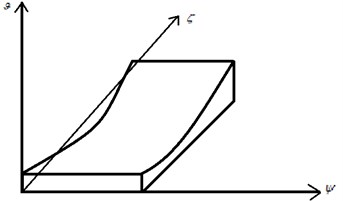Using Eq. (9), Eq. (10) becomes:

11
$E={E}_{0}\left[1-\alpha \left\{1-\frac{\zeta }{a}\right\}\left\{1-\frac{\psi }{b}\right\}\right],$

where $\alpha$, (0 ≤ $\alpha$ < 1) is called temperature gradient, which is the product of temperature at origin and slope of variation i.e., $\alpha =\gamma {\tau }_{0}$. For non homogeneous consideration in plate’s material, Poisson’s ratio of the plate varies exponentially in one direction as:

12
$\nu ={\nu }_{0}\left[{e}^{m\frac{\zeta }{a}}\right],$

where $m$, (0 ≤ $m$ ≤ 1) are known as non homogeneity constant.

Using Eqs. (8), (11) and (12), flexural rigidity of the plate becomes:

13
${D}_{1}={E}_{0}{{l}_{0}}^{3}\left[1-\alpha \left\{1-\frac{\zeta }{a}\right\}\left\{1-\frac{\psi }{b}\right\}\right]{\left[1+\beta \left(1-\sqrt{1-\frac{{\zeta }^{2}}{{a}^{2}}}\right)\right]}^{3}/12\left(1-{{\nu }_{0}}^{2}{e}^{2m\frac{\zeta }{a}}\right).$

## 4. Solution of the problem

We are using Rayleigh Ritz technique (i.e., maximum strain energy ${V}_{s}$ must equal to maximum kinetic energy ${T}_{s}$) in order to obtain frequency equation and frequencies for both modes of vibrations. Therefore, we must have:

14
$\delta \left({V}_{s}-{T}_{s}\right)=0.$

Here the expression for ${V}_{s}$ and ${T}_{s}$ are given by:

15
16

Here, we are computing frequency on C-C-C-C condition (i.e., all the four edges are clamped), therefore the boundary conditions are:

17

Therefore, deflection function (i.e, maximum displacement) which satisfy boundary condition given in Eq. (17) is taken as :

18
$\mathrm{\Phi }\left(\zeta ,\psi \right)={\left(\frac{\zeta }{a}\right)}^{2}{\left(\frac{\psi }{b}\right)}^{2}{\left(1-\frac{\zeta }{a}\right)}^{2}{\left(1-\frac{\psi }{b}\right)}^{2}\left[{\mathrm{\Omega }}_{1}+{\mathrm{\Omega }}_{2}\left(\frac{\zeta }{a}\right)\left(\frac{\psi }{b}\right)\left(1-\frac{\zeta }{a}\right)\left(1-\frac{\psi }{b}\right)\right],$

where ${\mathrm{\Omega }}_{1}$ and ${\mathrm{\Omega }}_{2}$ are arbitrary constants. On substituting Eqs. (8), (12) and (13) in Eqs. (15) and (16) we have:

19
${V}_{s}=\frac{{E}_{0}{{l}_{0}}^{3}}{24}\underset{0}{\overset{a}{\int }}\underset{0}{\overset{b}{\int }}\mathrm{‍}\left[\begin{array}{l}\left\{\frac{\left[1-\alpha \left\{1-\frac{\zeta }{a}\right\}\left\{1-\frac{\psi }{b}\right\}\right]{\left[1+\beta \left(1-\sqrt{1-\frac{{\zeta }^{2}}{{a}^{2}}}\right)\right]}^{3}}{1-{{\nu }_{0}}^{2}{e}^{2m\frac{\zeta }{a}}}\right\}\\ \bullet \left[{\left(\frac{{\partial }^{2}\mathrm{\Phi }}{\partial {\zeta }^{2}}\right)}^{2}+{\left(\frac{{\partial }^{2}\mathrm{\Phi }}{\partial {\psi }^{2}}\right)}^{2}+2{\nu }_{0}{e}^{m\frac{\zeta }{a}}\frac{{\partial }^{2}\mathrm{\Phi }}{\partial {\zeta }^{2}}\frac{{\partial }^{2}\mathrm{\Phi }}{\partial {\psi }^{2}}+2\left(1-{\nu }_{0}{e}^{m\frac{\zeta }{a}}\right){\left(\frac{{\partial }^{2}\mathrm{\Phi }}{\partial \zeta \partial \psi }\right)}^{2}\right]\end{array}\right]d\psi d\zeta ,$
20
${T}_{s}=\frac{1}{2}{\omega }^{2}\rho {l}_{0}\underset{0}{\overset{a}{\int }}\underset{0}{\overset{b}{\int }}\left[1+\beta \left(1-\sqrt{1-\frac{{\zeta }^{2}}{{a}^{2}}}\right)\right]{\mathrm{\Phi }}^{2}d\psi d\zeta .$

Now converting $\zeta$ and $\psi$ into non dimensional variable as:

21

Using Eq. (21), Eqs. (19) and (20) converted into:

22
23

Using Eqs. (22) and (23), Eq. (14) becomes:

24
$\delta \left({{V}_{s}}^{\mathrm{*}}-{\lambda }^{2}{{T}_{s}}^{\mathrm{*}}\right)=0,$

where ${\lambda }^{2}=12\rho {\omega }^{2}{a}^{4}/{E}_{0}{{l}_{0}}^{2}$ is known as frequency parameter. Eq. (24) consists of two unknown constants ${\mathrm{\Omega }}_{1}$ and ${\mathrm{\Omega }}_{2}$ (because of substitution of deflection function $\mathrm{\Phi }\left(\zeta ,\psi \right)$). These two unknowns could be calculated as follows:

25

After simplifying Eq. (25), we get system of homogeneous equations as:

26
$\begin{array}{l}{c}_{11}{\mathrm{\Omega }}_{1}+{c}_{12}{\mathrm{\Omega }}_{2}=0,\\ {c}_{21}{\mathrm{\Omega }}_{2}+{c}_{22}{\mathrm{\Omega }}_{2}=0.\end{array}$

To obtain non zero solution (frequency equation), the determinant of coffiecient matrix (symmetric matrix) of Eq. (26) must zero i.e.:

27
$\left|\begin{array}{ll}{c}_{11}& {c}_{12}\\ {c}_{21}& {c}_{22}\end{array}\right|=0.$

Eq. (27) is quadratic equation from which we get two modes as ${\lambda }_{1}$ (first mode) and ${\lambda }_{2}$ (second mode).

## 5. Results and discussion

The first two modes of vibrations are calculated and presented tabularly as well as graphically for different values of thermal gradient $\alpha$, non homogeneity constant $m$ and tapering parameters $\beta$. The parameters used in numerical calculation are ${E}_{0}=$ 7.08×1010 nm-2, ${\nu }_{0}=$ 0.345, ${l}_{0}=$ 0.01 m, $\rho =$ 2.80×103 kgm-3.

Table 1 provides the natural vibration (first two modes) of plate corresponding to thermal gradient$\alpha$ for three different combination of non homogeneity $m$ and taper constant$\beta$ i.e., $m=\beta =$ 0.2, $m=\beta =$ 0.4 and $m=\beta =$ 0.8. From Table 1, we can see that frequency of both modes decreases with the increasing value of thermal gradient $\alpha$ for all the combination of non homogeneity $m$ and taper constant $\beta$. While the frequency modes of vibration increases with the increasing value of thermal gradient $\alpha$ when the combined value of non homogeneity $m$ and taper constant $\beta$ varies from 0.2 to 0.8.

Table 2 shows the frequency modes of vibration corresponding to non homogeneity constant $m$ for the following values of taper constant $\beta$ and thermal gradient $\alpha$. $\alpha =\beta =$ 0.2, $\alpha =\beta =$ 0.4 and $\alpha =\beta =$ 0.8.

Table 2 provides the fact that vibrational frequency increases rapidly (not in linear fashion) when non homogeneity $m$ in plate’s material increases 0 to 1 for all the above mentioned values of thermal gradient $\alpha$ and tapering parameter $\beta$. The vibrational frequency also increases when the combined value of thermal gradient $\alpha$ and tapering parameter $\beta$ varies from 0.2 to 0.8 with the increasing value of non homogeneity constant $m$.

Table 1Thermal gradient α vs vibrational frequency λ for a/b= 1.5

 $\alpha$ $\beta =m=$ 0.2 $\beta =m=$ 0.4 $\beta =m=$ 0.8 ${\lambda }_{1}$ ${\lambda }_{2}$ ${\lambda }_{1}$ ${\lambda }_{2}$ ${\lambda }_{1}$ ${\lambda }_{2}$ 0.0 68.16 268.66 72.15 283.85 82.92 327.10 0.2 66.50 262.09 70.45 277.19 81.14 320.21 0.4 64.79 255.36 68.72 270.37 79.31 313.16 0.6 63.04 248.45 66.94 263.37 77.44 305.96 0.8 61.24 241.45 65.11 256.18 75.52 298.58

Table 2Non homogeneity m vs vibrational frequency λ for a/b= 1.5

 $m$ $\alpha =\beta =$ 0.2 $\alpha =\beta =$ 0.4 $\alpha =\beta =$ 0.8 ${\lambda }_{1}$ ${\lambda }_{2}$ ${\lambda }_{1}$ ${\lambda }_{2}$ ${\lambda }_{1}$ ${\lambda }_{2}$ 0.0 65.51 258.12 66.35 260.63 68.24 266.63 0.2 66.50 262.09 67.36 264.79 69.30 271.18 0.4 67.81 267.38 68.72 270.37 70.75 277.43 0.6 69.55 274.51 70.55 278.00 72.75 286.16 0.8 71.91 284.31 73.04 288.60 75.52 298.58 1.0 75.10 297.96 76.45 303.56 79.34 316.50

Table 3 gives the frequency modes corresponding to thickness variation (tapering parameter $\beta$) in plate for the following values of non homogeneity constant $m$ and thermal gradient $\alpha$. $m=\alpha =$ 0.2, $m=\alpha =$ 0.4 and $m=\alpha =$ 0.8.

Form Table 3, one can easily concludes that when tapering parameter $\beta$ increases from 0 to 1, frequency modes increases for all the above mentioned values of non homogeneity $m$ and thermal gradient $\alpha$. The frequency modes decrease when the combined value of non homogeneity $m$ and thermal gradient $\alpha$ varies from 0.2 to 0.4. But when the combined value of non homogeneity $m$ and thermal gradient $\alpha$ varies from 0.4 to 0.8, the frequency modes again increases.

In order to get good understanding of results and discussion (variation of plate parameter), graphical representation of Tables 1-3 are presented in the form of Figs. 2-4.

Table 3Taper constant β vs vibrational frequency λ for a/b= 1.5

 $\beta$ $m=\alpha =$ 0.2 $m=\alpha =$ 0.4 $m=\alpha =$ 0.8 ${\lambda }_{1}$ ${\lambda }_{2}$ ${\lambda }_{1}$ ${\lambda }_{2}$ ${\lambda }_{1}$ ${\lambda }_{2}$ 0.0 64.07 253.24 63.58 251.36 63.61 252.08 0.2 66.50 262.09 66.07 260.57 66.38 262.69 0.4 69.08 271.53 68.72 270.37 69.30 274.01 0.6 71.78 281.50 71.49 280.73 72.35 285.99 0.8 74.61 291.98 74.39 291.63 75.52 298.58 1.0 77.55 302.94 77.39 303.01 78.79 311.73

## 6. Results comparison

A comparison of present analysis with [11, 12] are presented in tabular (Table 4 and Table 5) as well as in graphical form (Fig. 5 and Fig. 6).

Fig. 2Thermal gradient (α) vs. frequency (λ) for fixed a/b= 1.5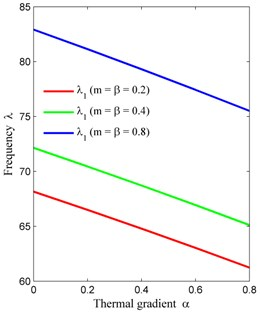a)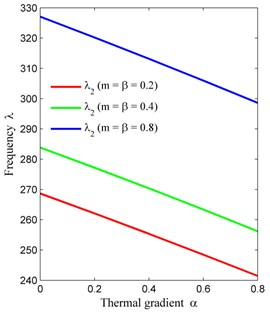b)

Fig. 3Non homogeneity constant (m) vs. frequency (λ) for fixed a/b= 1.5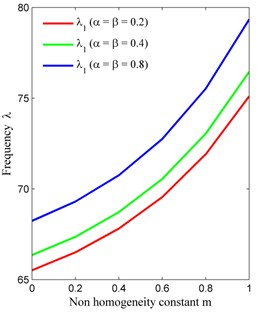a)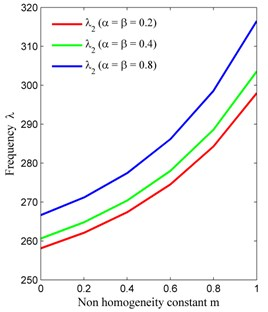b)

Fig. 4Taper constant (β) vs. frequency (λ) for fixed a/b= 1.5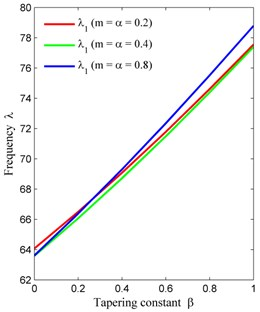a)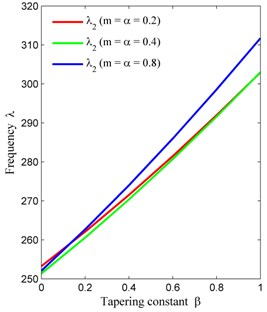b)

Table 4 shows the comparison of frequency modes of present paper with [11, 12] corresponding to tapering parameter $\beta$ for fixed value of thermal gradient $\alpha$ and non homogeneity constant $m$ i.e., $\alpha =m=$ 0. Table 4 provides the information that frequency modes in present paper are less when compared with [11, 12]. The frequency modes of present paper, [11, 12] are coincides at $\alpha =m=\beta =$ 0.

Fig. 5Comparison of frequency modes with [11, 12] corresponding to taper constant (β)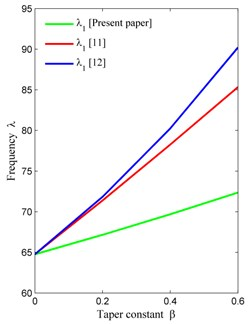a)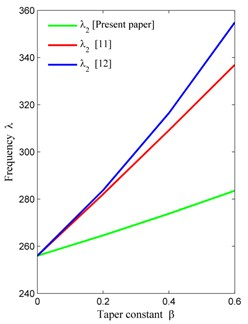b)

Fig. 6Comparison of frequency modes with  corresponding to thermal gradient (α)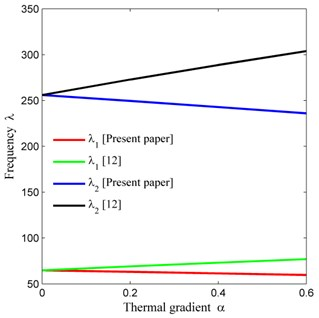Table 4Comparison of frequency modes of presented study with [11, 12] corresponding to taper constant β

 $\beta$ $m=\alpha =$ 0.0 ${\lambda }_{1}$ ${\lambda }_{2}$ 0.0 64.77 255.98 64.77 255.98 64.77 255.98 0.2 71.40 282.12 67.16 264.61 71.84 283.76 0.4 78.27 309.17 69.69 273.81 80.25 316.46 0.6 85.34 336.90 72.36 283.53 90.21 354.87 Note: The value written in bold and italic are from [11, 12] respectively

Table 5 portrays the comparison of frequency modes of present paper with  corresponding to thermal gradient $\alpha$ for fixed value of tapering parameter $\beta$ and non homogeneity constant $m$ i.e., $m=\beta =$ 0. It is noticed from Table 5 that frequency modes of present paper are less when compared with . Frequency modes of present paper and  are coincides at $\alpha =m=\beta =$ 0.

Table 5Comparison of frequency modes of present study with  corresponding to thermal gradient α

 $\alpha$ $\beta =m=$ 0.0 ${\lambda }_{1}$ ${\lambda }_{2}$ 0.0 64.77 255.98 64.77 255.98 0.2 63.13 249.50 69.09 272.86 0.4 61.45 242.84 73.10 288.75 0.6 59.71 236.00 76.92 303.82 Note: The value written in bold are from 

## 7. Conclusions

From the results discussion and comparison authors would like to record the following points.

1) The frequency modes are less in case of circular variation in thickness (present paper) when compared to linear variation in thickness  and exponential variation in thickness  as shown in Table 4 and Fig. 5.

2) The frequency modes are less in case of bi linear temperature variation on plate (present paper) when compared to exponential variation in temperature on plate  as shown in Table 5 and Fig. 6.

3) The frequency modes decrease when the temperature increases on the plate as shown in Table 1 and Fig. 2.

4) When the non homogeneity increases in plate materials, frequency modes increases as shown in Table 2 and Fig. 3.

5) The frequency modes also increase when the thickness of plate increases as shown in Table 3 and Fig. 4.

#### Cited by

Thermal Vibration of Rectangle Plate with Two-Dimensional Circular Thickness Effect